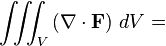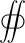# Verify Divergence Theorem

nestleeng

## Homework Statement

Verify the divergence theorem for the function
V = xy i − y^2 j + z k
and the surface enclosed by the three parts
(i) z = 0, s < 1, s^2 = x^2 + y^2,
(ii) s = 1, 0 ≤ z ≤ 1 and
(iii) z^2 = a^2 + (1 − a^2)s^2, 1 ≤ z ≤ a, a > 1.

## Homework Equations

[/B]Divergence theorem, although on the RHS I put vector DS = nDS.

## The Attempt at a Solution

So I solved the LHS and got the answer to be a*Pi

on the RHS, splitting the 3 surfaces,
(i) got 0 for integral
(ii) got 0 for integral
(iii) staying in cartesians, I have to integrate ((1-a^2)(-x^2 y +y^3 + x^2 +y^2) +a^2)/Sqrt(a^2+(1-a^2)(x^2+y^2) dxdy between -1 and 1 for x and y which even Wolfram can't do.

You haven't really said much about what you did. What did you get for $\nabla\cdot\vec{V}$? What are s and a? Constants? Then "(ii) s= 1, $0\le z\le 1$" makes no sense.